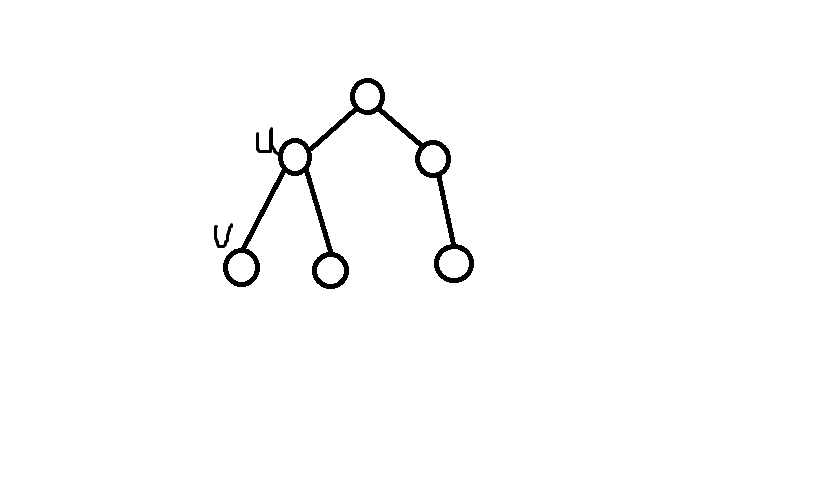# Highway

In ICPCCamp there were  n  towns conveniently numbered with  1,2,,n  connected with  (n1)  roads. The  i -th road connecting towns  ai  and  bi  has length  ci . It is guaranteed that any two cities reach each other using only roads.

Bobo would like to build  (n1)  highways so that any two towns reach each using only highways. Building a highway between towns  x  and  y  costs him  δ(x,y)  cents, where  δ(x,y)  is the length of the shortest path between towns  x  and  y  using roads.

As Bobo is rich, he would like to find the most expensive way to build the  (n1)  highways.

## Input

The input contains zero or more test cases and is terminated by end-of-file. For each test case:

The first line contains an integer  n . The  i -th of the following  (n1)  lines contains three integers  ai bi  and  ci .

• 1n105
• 1ai,bin
• 1ci108
• The number of test cases does not exceed  10 .

## Output

For each test case, output an integer which denotes the result.

## Sample Input

5
1 2 2
1 3 1
2 4 2
3 5 1
5
1 2 2
1 4 1
3 4 1
4 5 2


## Sample Output

19
15

dp[u]:u到子树节点的最长距离，dp[u]:u到子树节点外其他节点的最长距离

dp[u]很容易在dfs中计算出来。dp[v]:若v不是u子树最长路径经过的点，dp[v] = max( dp[u]+Luv , dp[u]+Luv )

若v是u子树最长路径所经过的点，dp[v] = max( dp[u]+Luv , dp[u]+Se[u])

Se[u]带表u的子树中第二长的路径长度。

#include <cstdio>
#include <cstring>
#include <cmath>
#include <iostream>
#include <queue>
#include <set>
#include <string>
#include <stack>
#include <algorithm>
#include <map>
using namespace std;
typedef  __int64 ll;
const int N = 100100;
const int M = 20;
const int INF = 0x3fffffff;

struct  Edge
{
int node,len;
Edge*next;
}m_edge[N*2];
int Ecnt;
int vis[N],flag[N];
ll dp[N],second[N];
//dp[u]:u到子树节点的最长距离,dp[u]:u到子树节点外其他节点的最长距离
//second[u]:子树节点到u的第二长路径，flag[v]:节点v的上层节点的最长路径是否经过v

void init()
{
Ecnt = 0;
fill( flag , flag+N , 0 );
fill( second , second+N , 0 );
}

void mkEdge( int a , int b , int c )
{
m_edge[Ecnt].node = a;
m_edge[Ecnt].len = c;

m_edge[Ecnt].node = b;
m_edge[Ecnt].len = c;
}

ll dfs1( int u )
{
vis[u] = true; dp[u] = 0;
for( Edge*p = head[u] ; p ; p = p->next ){
int v = p->node;
if( !vis[v] ){
ll w = dfs1( v );
dp[u] = max( dp[u] , w+p->len );
}
}
return dp[u];
}

void dfs2( int u  )
{
vis[u] = true;
int fg = 0;
for( Edge*p = head[u] ; p ; p = p ->next ){
int v = p->node;
if( !vis[v] ){
if( !fg && dp[u] == dp[v]+p->len ) { flag[v] = 1;fg = 1; }
else second[u] = max( second[u] , dp[v]+p->len );
dfs2( v );
}
}
}

void dfs3( int u )
{
vis[u] = true;
for( Edge*p = head[u] ; p ; p = p->next ){
int v = p->node;
if( !vis[v] ){
if( !flag[v] ) dp[v] = max( dp[u]+p->len , dp[u]+p->len );
else dp[v] = max( dp[u]+p->len , second[u]+p->len );
dfs3( v );
}
}
}

int main()
{
int n,a,b,c;
while( ~scanf("%d",&n) ){
init();
for( int i = 0 ; i < n-1 ; ++i ){
scanf("%d%d%d",&a,&b,&c);
mkEdge( a , b , c );
}
memset( vis , 0 , sizeof(vis) );
dfs1( 1 );
memset( vis , 0 , sizeof(vis) );
dfs2( 1 );
memset( vis , 0 , sizeof(vis) );
dfs3( 1 );
ll rec = 0,ans = 0;
for( int i = 1 ; i <= n ; ++i ){
ll t = max( dp[i] , dp[i] );
ans += t;
rec = max( rec , t );
}
ans -= rec;
printf("%I64d\n",ans);
}
return 0;
}

### Java中解析日志_碧海凌云的博客-程序员信息网_java 日志解析

Java中解析日志常用的是Grok，Grok是一个用于解析logs和其它文件的简单易用的API，并且它可以将这些无结构的logs转换成结构化的数据（JSON）,使用正则表达式对logs进行解析，常用的是grok和java-grok，下面来具体说说各自的使用grokgrok是io.thekraken组织提供的实现，在mven仓库中输入io.thekraken就可以找到对应的jar包简单Java项目如果是简单Java项目，直接引入依赖的jar包即可，对于0.1.5的版本，必须引入5个jar包：comm

### Linux服务 Nginx(一)_dingcong4061的博客-程序员信息网

Linux服务 Nginx(一) Nginx是一款轻量级的Web服务器/反向代理服务器及电子邮件（IMAP/POP3）代理服务器，并在一个BSD-like 协议下发行.其特点是占有内存少，并发能力强，事实上nginx的并发能力确实在同类型的网页服务器中表现较好，中国大陆使用nginx网站用户有：百度、京东、新浪、网易、腾讯、淘宝等. http协议...

### Java AES加密案例_diaomu5377的博客-程序员信息网

AES加密原理http://www.blogjava.net/amigoxie/archive/2014/07/06/415503.htmlPHP 加密https://segmentfault.com/a/1190000002798716Java AES接口详解http://blog.zheezes.com/java-aes-encryption-uses-and-p...